# OECD Discriminatory family code data analysis 4 - Bootstrap method for getting Confidence Interval with RPhoto by Jeremy Santana on Unsplash

This post is following of above post.
In the previous post, I get Confidence Interval using standard error.

In this post, I will get Confidence Interval using Bootstrap method.

Bootstrap method is to draw random samples many times and calclates sample averages and get quantile value.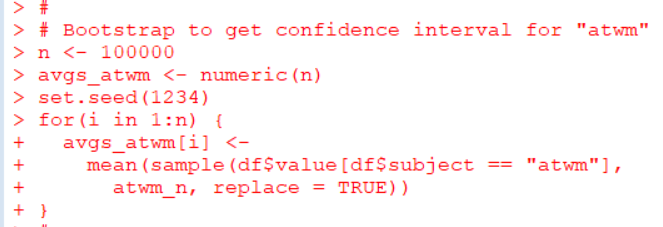I draw 100,000 samples and calculate average 100,000 times. I use for() loop.

Those 100,000 averages are store in avgs_atwm object.

Let's see a histogram of avgs_atwm.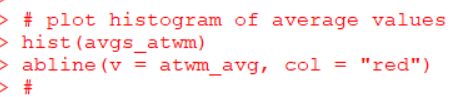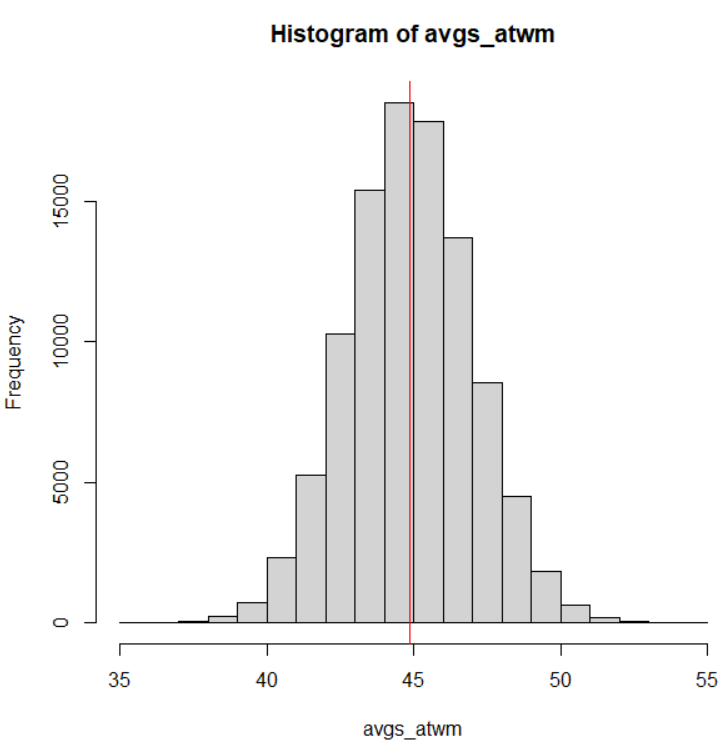Vertical red line is an average of avgs_atwm.

Then, I use quantile() function to get 2.5% quantile and 97.5% quantile values.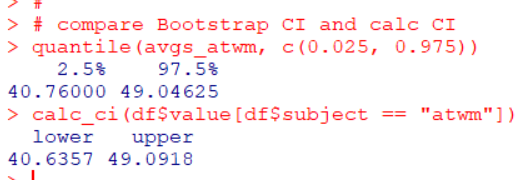Bootstrap confidence interval is 40.76 ~ 49.04 while standard error confidence interval is 40.64 ~ 49.09. The both are quite similar value.

Next, let's do with "em"; Early Marriage.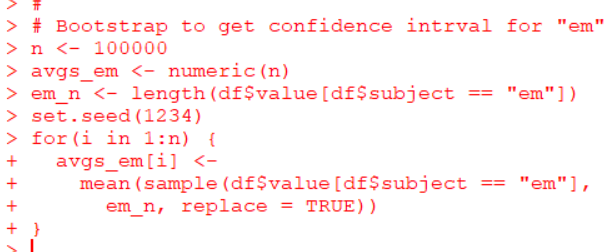Make a histogram.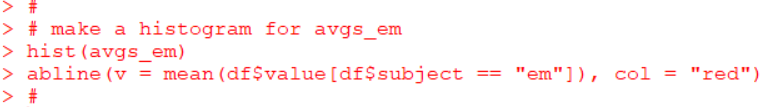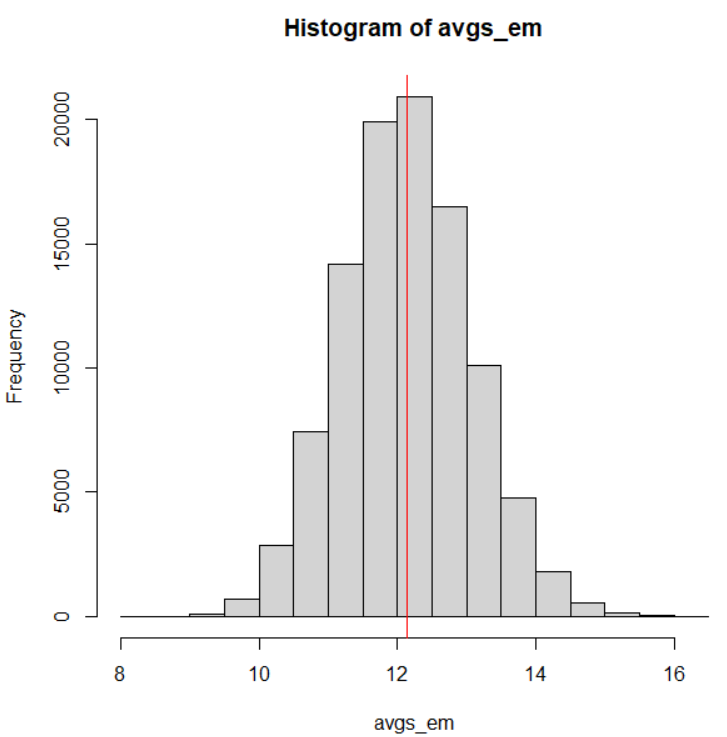Let's compare Bootstrap Confidence Interval and Standard Error Confidence Interval.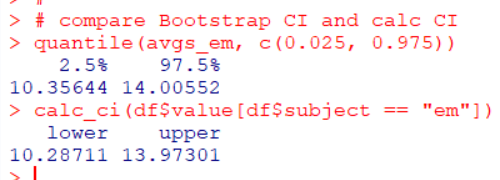The both CI are quite similar.

That's it. Thank you!

Next post is